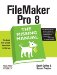# Number Crunching Calculations

Although they don't come first in the function list, number functions are the most obvious application of calculations. Since most people easily understand them, number functions are a logical place to start. A lot of the concepts you'll learn for number functions apply to other functions as well.

10.1.1. Number Operators

Operators, along with fields, constants, and functions, are one of the basic building blocks of a calculation. FileMaker has five operators that specifically apply to numerical calculations, the same five that you probably already know:

• - is for subtraction
• * is for multiplication
• / is for division
• ^ is for exponentiation ("to the power of")

Section 9.2.2.2 explains how to use these operators in detail. In your day-to-day work with FileMaker, you usually use these operators to calculate the numerical values in your fields.

Note: If you're new to calculations, you'll want to get a handle on the material in this chapter before proceeding. But if you've worked with logical tests before, you can skip straight to Chapter 11.

Say you're an event planner and you always plan for 10 percent above the expected number of attendees. Use this calculation to find your fudge factor:

```	Sum ( Attendees::Confirmed ) * 1.1
```

10.1.2. Number Function Types

FileMaker also gives you dozens of functions that do special things to numbers. Because there are so many number functions, the function list breaks them up into smaller groups. The groups have descriptive names, so you can drive right to the group you need (or skim by them without looking, if you might be traumatized if you accidentally saw a sine or cosine function). The functions you use with your numeric data are:

• Number functions
• Aggregate functions
• Financial functions
• Trigonometric functions

As you saw on Section 9.2.4, every number function expects one or more parameters, each a number value, and all the number functions return a number result. Here's how to put them to work:

Note: This book doesn't cover the financial and trigonometric functions, which have highly specialized uses. If you need to use these brawny functions, you probably have the mental muscle to decipher the technical terms in FileMaker's Help file, where you'll find them explained.

10.1.3. Precision

Some calculations demand a high degree of precision, like those that track radioactive isotopes or other scientific data with lots of places following the decimal point. FileMaker comes prepared with the SetPrecision function. This function extends FileMaker's default precision of 16 decimal places, up to a maximum of 400. If you need more precision, you add a SetPrecision function to the calculation that produces the value that requires precision. You can use SetPrecision with all other numeric functions, except trigonometric functions, which don't accept this extended precision. See Figure 10-1.Figure 10-1. The Default Result field shows the result of dividing one by three. In the field called Precise Result, the calculation is changed to use the SetPrecision function (as you can see in the background window). This version gives a more precise answer.

The SetPrecision function requires two parameters. The first parameter is a number, or any expression that results in a number. The second is the number of decimal places you want to see.

This calculation would return Pi with 3 decimal places:

```	SetPrecicion( Pi ; 3 )
```

This version would show 100 decimal places:

```	SetPrecision( Pi ; 100 )
```

Note: The SetPrecision function affects your calculation's result, but not the way FileMaker displays it. To save space on your layout, you can format a very precise calculation to show only a few decimal places until you click the field, when you see the stored value (Figure 10-1).FileMaker Pro 8: The Missing Manual
ISBN: 0596005792
EAN: 2147483647
Year: 2004
Pages: 176